### Quadrilateral Inscribed In A Circle

An illustration showing a quadrilateral inscribed in a circle that is tangent to a line.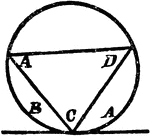### Triangle Inscribed In A Circle

An illustration showing a triangle with angles A, C, and D inscribed in a circle which is tangent to…### Parallel Tangents to an Ellipse

Illustration showing that tangents drawn at the ends of any diameter are parallel to each other.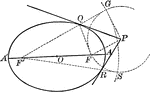### Tangent From External Point to an Ellipse

Illustration of how to draw a tangent to an ellipse from an external point.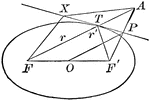### Tangent to an Ellipse

Diagram an ellipse with a tangent line that illustrates "A line through a point on the ellipse and bisecting…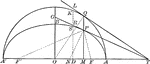### Tangents to an Ellipse

Illustration showing the tangents drawn at two corresponding points of an ellipse and its auxiliary…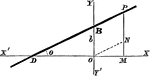### Line Equation

To find the equation of a straight line in "tangent" form.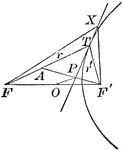### Tangent to a Hyperbola

Diagram part of a hyperbola with a tangent line that illustrates "A line through a point on the hyperbola…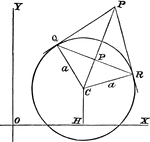### Tangent Length

Finding the length of a tangent from a given point to a circle.